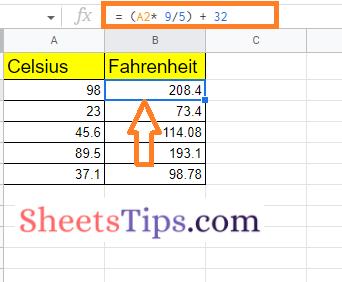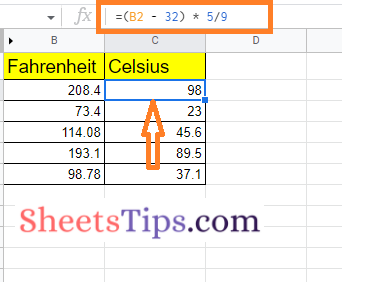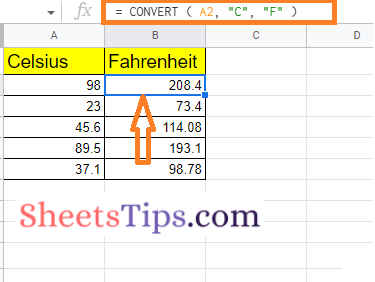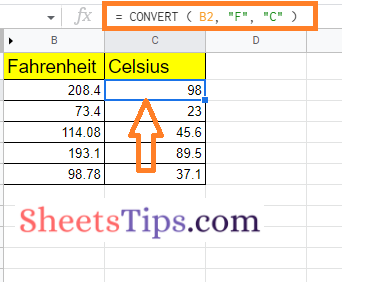# How to Convert Temperatures from Celsius to Fahrenheit in Google Sheets Using Examples

When working with a dataset that contains temperature values, it is quite common to want to convert the Celsius data to Fahrenheit. The conversion of Celsius to Fahrenheit is important since a few countries follow temperature as a standard measure, while a few others follow Fahrenheit as their standard measure.

## How Do You Change Temp From C to F Using the Basic Formula?

The basic formula to convert Celsius to Fahrenheit is F = (C * 9/5) + 32. So let us now incorporate this function into Google Sheets. The steps to convert Celsius to Fahrenheit using basic formulas with the help of the steps outlined below

• 2nd Step: Now on the homepage, move to the cell where you want to get the conversion results.
• 3rd Step: Enter the formula “= (A2*9/5)+32“.
• 4th Step: Press the “Enter” key and you will find the results as shown in the image given below.### How Do I Change From Farenheit to Celcius in Google Sheets?

The basic formula to convert Farenheit to Celcius is C = (F-32) * 5/9. The steps to convert Fahrenheit to Celcius in Google Sheets using this basic formula are given below.

• 2nd Step: Now on the homepage, click on the cell where you want to get the results of F to C.
• 3rd Step: In the specified cell, enter the formula “= (B2-32)*5/9“.
• 4th Step: Press the “Return” key and you will find the results as shown in the image given below.### Using the CONVERT Function to Convert Celsius to Fahrenheit in Google Sheets

One of the most useful functions to convert Celsius to Fahrenheit in a spreadsheet is the CONVERT function. The steps to using the CONVERT function to change Celsius to Fahrenheit in the spreadsheet are outlined below.

• 2nd Step: Move to the cell where you want to show the conversion results.
• 3rd Step: Here enter the formula “= CONVERT (A2, “C”, “F”)“.
• 4th Step: Press the Enter key and you will find the results as shown in the image given below.Formula Explanation

The start unit “C” indicates the Celsius temperature scale and assumes the value contained in cell A2 is in Celsius.

The end unit parameter is set to “F,” indicating to the system that the conversion should be in Fahrenheit.

### Using the CONVERT Function to Convert Fahrenheit to Celsius in Google Sheets

The steps to change the measurement value from Fahrenheit to Celsius in the Google Spreadsheet are outlined below.